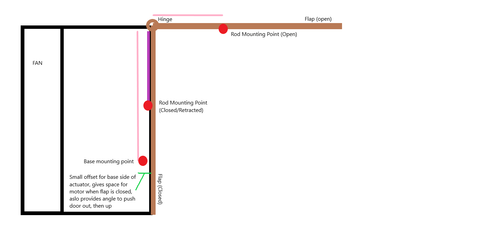# What If My Hinge Is On Top? Technical Guide with Illustrations

One of the more difficult applications to grapple with is the “top-hinged flap” application; sometimes referred to (by me) as the “garage door design”. For purposes of explanation, we will be using terminology laid out in this article only.

### Before we begin:

There are calculators out there that can help with this application; most will not explain how they work, they will just spit out a numerical answer (or product suggestion). At time of posting, Our 'top hinge' calculator is just a spreadsheet with equations. This article is more about the how. We hope it helps you gain further insight into your project.

While this application looks intimidating in concept, it can be broken down into some quick trigonometry and a lever-based calculation, both of which can be done easily with a calculator or the internet. For this article I will be using the example of an attic fan-cover that a customer made previously. The fan was in the wall, leading to the outside, but that also meant heat could escape too easily during the cold seasons; so the owner wanted an insulated cover to help maintain heat in the winter, but allow for easy ventilation in the summer (without climbing into the attic to flip the flap!).

To start, we only need the weight of your hinged-flap (total weight) and the length from the hinge to the opposite edge. There will be some math ahead.The labelled illustration above shows the required dimensions for the application (without actual measurements, in this case). The important lengths are the Rod Mounting Point on the Flap, which should be around 20%-30% of the distance from the hinge to the edge of the moving door.

First, lets define the mounting points and understand why they must be just so; The Rod Mounting Point will be about ¼ of the distance from the hinge to the edge, if the Rod Mounting Point is too far in, the force required from the actuator will rise significantly, if it is too far out, the actuator stroke will not fit correctly with the application, leading to a less-than 90 degree lift. This point is an approximation of the ratio for a happy-medium between the two.

## Part One: Forcing It

The first calculation we should make is to determine the force on the actuator. You may recognize this application as a Class 3 Lever (like a fishing pole or broom) - the load is on one end, the hinge is opposite, and the effort load is in between the two.

This arrangement requires a higher effort force from the actuator, but means that the flap moves further with less motion (higher force, lower stroke requirement).

The Equation for this Simple Lever Operation is:

or

### -(Force) = (Load * Length)/(Mounting Distance)

also expressed with variables as:

### -F=(L*D)/(M)

Now, plugging in some numbers into the equation; for this example lets say the flap is 100 pounds, and 30" long.

-F = (100# * 30") / (6")
-F= 3000/6
-F= 500 lbs.

As you can see, this type of lever requires a higher load actuator. This does not mean you will need a 500 pound actuator, it means you will need a more than 500 pound actuator force.

## Part Two: Finding Your Specifications

First, figuring out the necessary distances; this would be where you expect to dust off your high school trigonometry textbook, but you won't need to. We would be using this only to find the ideal actuator stroke length for the application. Which means we will only need the one equation nestled deep in your subconscious - the Pythagorean theorem.

A^2 + B^2 = C^2 where A = Flap Mount Position, B= the Wall Mounting Position, and C = Your Actuator's Extended Length.

### Hang on, we only have one of these figured out!

Correct, now it's time for a little estimate and check. Take your time perusing our website, check the Specifications and Technical Drawings of the actuators that you think might work - For my example, and as a general rule of thumb, I will use the Premium Actuator line. Due to the extensive stroke length options and the weight ratings reaching all the way to 400 pounds, these are almost always the best 'standardized' starting point to use for estimations.

Following the above instructions; a 12" Premium Actuator has an extended length of around 28", which is the longest possible stroke without over-extending our door flap, or under-moving the door. We will plug this extended actuator length into our Pythagorean Theorem as C.

A^2 + B^2 = C^2
where A = 6", B = Wall Mounting Position, and C = ~28.

(28^2) - (6^2) = B^2
784 - 36 = 748
sqrt(748) = 27.349... = Estimated Wall Mounting Position (about 27.3")

## Putting It All Together

Below is the diagram you've seen previous with some added illustration; This shows that the length of the actuator changes only slightly by adding a 1-2" offset on the base end of the unit (the Wall Mount Position)Using our length solution from part 2, we know the approximate length of the actuator we are considering - however, the weight requirement from part 1 is higher than the actuator we estimated for. How do we solve this? The easiest option is to use 2 actuators, splitting the load requirement per actuator in half. This would allow us to use 2x 400 Lbs Premium Line Actuators (including Optical Units). If your application cannot include two actuators, you would need to find an actuator that exceeds the weight requirement from part 1, but stays close to the length requirement from part 2. For this example, a Bullet .50 Cal 8" unit at 674 pounds or higher would work for this application.

That was a ﻿lot﻿ to consider; but it only needs to be done once; now that you know your specifications and have a general idea of which actuator you will need, you're ready to start planning your FIRGELLI system.